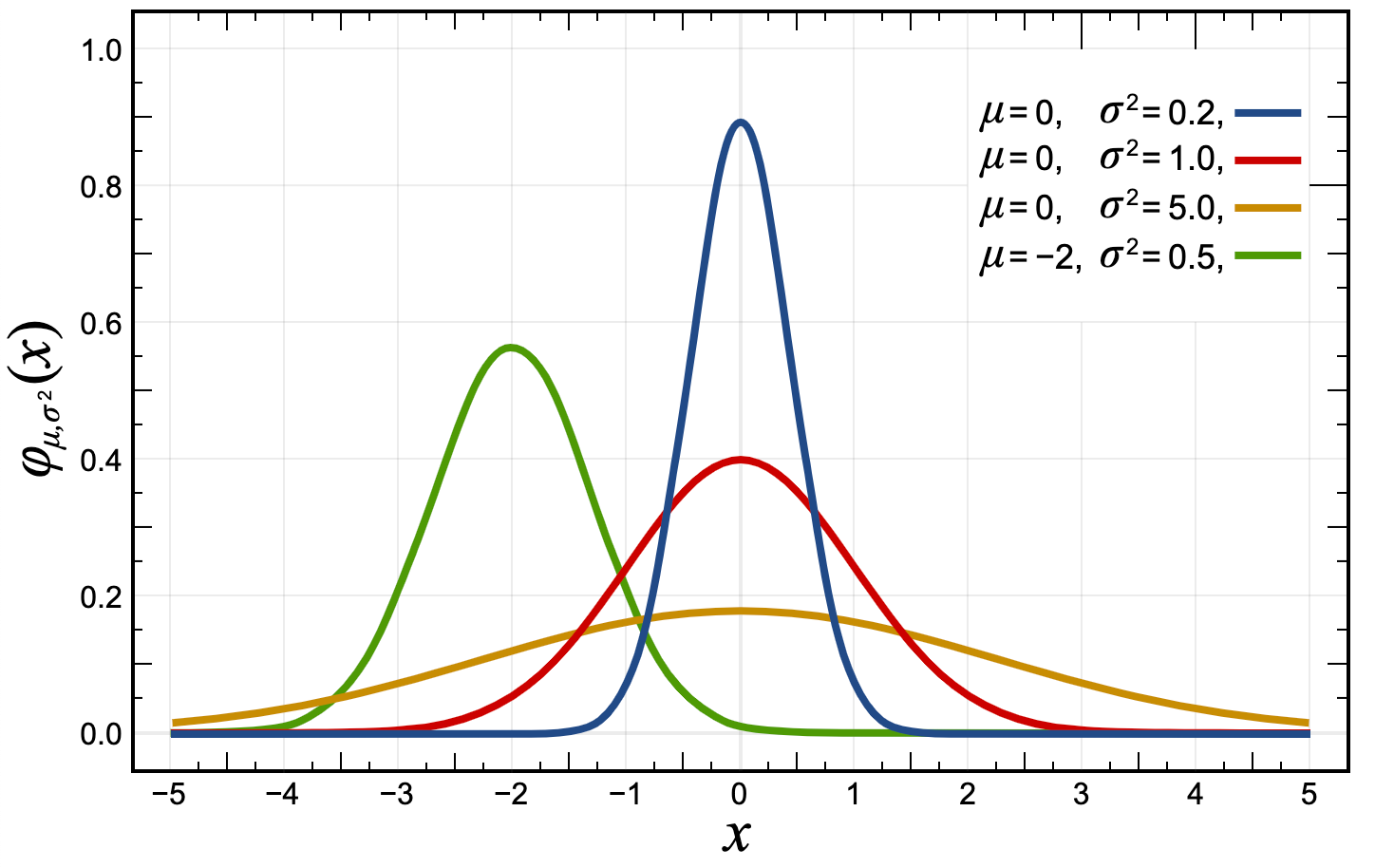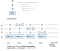# Preparing a Gaussian wave function in Q#

Happy day 19 of the Q# Advent Calendar! In this blog post, Andy Sun and I will talk about a small project we’ve been working on in the past few weeks.

The context of this project is what we call a “mini-mentorship”, where a member of the Microsoft Quantum team mentors someone from the Quantum community to produce an open source contribution to one of our QDK (Quantum Dev Kit) repositories. Andy has been working on a contribution to our Quantum samples repo — you can check out his code on GitHub, and soon in our official repo.

An interesting application for quantum computers that Andy has been working on for one of his PhD projects is the simulation of continuous physical systems (ref. , ). A number of near-term quantum applications currently focus on variational algorithms, meaning algorithms that modify an initial quantum state iteratively until a final state is reached that represents the solution. An important initial state for such an algorithm is the Gaussian wave function. We thought that preparing such a Gaussian would make a great introductory Q# sample.The normal distribution for a few different values of μ and σ. The red curve is also known as the “standard normal distribution”.

As you might probably (no pun intended) know, a Gaussian can be described by its standard deviation (σ) and mean (μ). To prepare a Gaussian state using a qubit register, what we do is basically prepare a large entangled state where the probability of achieving each bitstring corresponds to the value of the Gaussian for that particular value. So, to be more precise, for a given qubit register of size N, we want to prepare a state that has a probability distribution over all possible 2ᴺ bitstrings that looks like a Gaussian.

The algorithm Andy has chosen to use will achieve this by applying controlled rotations, starting with qubit at index 0, in a recursive manner. Mainly, this algorithm consist of the following steps (see ref. ):

1. Calculate a rotation angle α, computed using a desired standard deviation (σ) and mean (μ).
2. Apply the rotation R(α) to the qubit at index 0.
3. Compute σ₁ and μ₁, where σ₁=σ₀/2 and μ₁=μ₀/2 if the previously rotated qubit is |0〉, and μ₁=(μ₀-1)/2 if it is |1〉. This is a controlled rotation, so this happens quantum coherently.
4. On the remaining N-1 qubits of the register, apply steps 1–4.

The rotation angle α is defined as (ref. ):

α = cos⁻¹√(f(σ/2, μ/2)/f(σ, μ))

where

f(σ, μ) = Σ[n=-∞,∞] exp((n-μ)²/σ²)

So, now you might wonder, how do we implement a recursive quantum algorithm? We’re going to need a quantum language, such as Q#, that supports defining an operation and calling itself recursively. However, most common quantum programming languages don’t support this, and it’s also not the easiest to debug. So, as a first stab at implementing this, we’re going to take a brute-force approach and implement it using for-loops. For inspiration, we’re going to look at the implementation by M. Sohaib Alam in his blog post (ref. ).

In the blog post, the author implements the algorithm using such a for-loop approach and for each iteration, calculates the effective rotation angle θ using the above definition for α and the effective σ and μ that are modified as a consequence of step (3). As described in the algorithm, θ will be different for each iteration and will depend on the state of the control qubits in the register. I will explain later what this means and how we can implement that in Q#.

The effective rotation angles that depend on the control register can be described as follows for each iteration (ref. ):

(iteration n=0)

|0〉→ θ = α(σ, μ)

(iteration n=1)

|00〉→ θ = α(σ/2, μ/2)

|01〉→ θ = α(σ/2, (μ-1)/2)

(iteration n=2)

|000〉→ θ = α(σ/4, μ/4)

|001〉→ θ = α(σ/4, (μ-1)/4)

|010〉→ θ = α(σ/4, ((μ/2)-1)/2)

|011〉→ θ = α(σ/4, (((μ-1)/2)-1)/2)

From this, we can define an implementation of a function that returns the effective rotation angle for a given σ, μ and bitstring.

The main difference between how this blog post implements the algorithm and how we’ll implement it in Q#, however, is that on real hardware, we typically don’t have access to creating any arbitrary gate from a unitary matrix. In Q#, we will use a sequence of controlled rotation gates Ry, which is slightly closer to what might be available on quantum hardware.

We can express this in Q# by using the ApplyControlledOnBitString operation. This operation has the following signature:

operation ApplyControlledOnBitString<’T> (bits : Bool[], oracle : (‘T => Unit is Adj + Ctl), controlRegister : Qubit[], targetRegister : ‘T) : Unit is Adj + Ctl

Now, as previously mentioned, for every iteration through the qubit register, we will have a different effective rotation angle θ that depends on the control register. If we draw this in a circuit representation:

These are not “regular” controlled Ry gates. The (*) symbols indicate there is something special going on; for a given qubit N, the controlled gate will apply a given θ that is specific per bitstring of the previous N-1 qubits in the register. As drawn below:Example of a concrete circuit diagram representation of the controlled rotation as implemented by the ApplyControlledOnBitstring Q# operation for iteration n=3.

The “oracle” we use in the ApplyControlledOnBitstring operation is the controlled Ry(θ) operation, where θ depends on the qubit number and bitstring.

To see Andy’s implementation of the algorithm, check out his fork of the Quantum repo on GitHub, in the samples/Gaussian_initial_state folder. If you’d like to take on the challenge of implementing this algorithm recursively using Q#, please share your code and/or ideas with us in the comments or on the Q# community Slack.

We hope you enjoyed this explanation of how to create a Gaussian state using qubits in Q#. Happy Quantum Programming, happy holidays and wishing you all the best for |2021〉!

References:

Software Dev @ Microsoft Quantum

## More from Guen P

Software Dev @ Microsoft Quantum